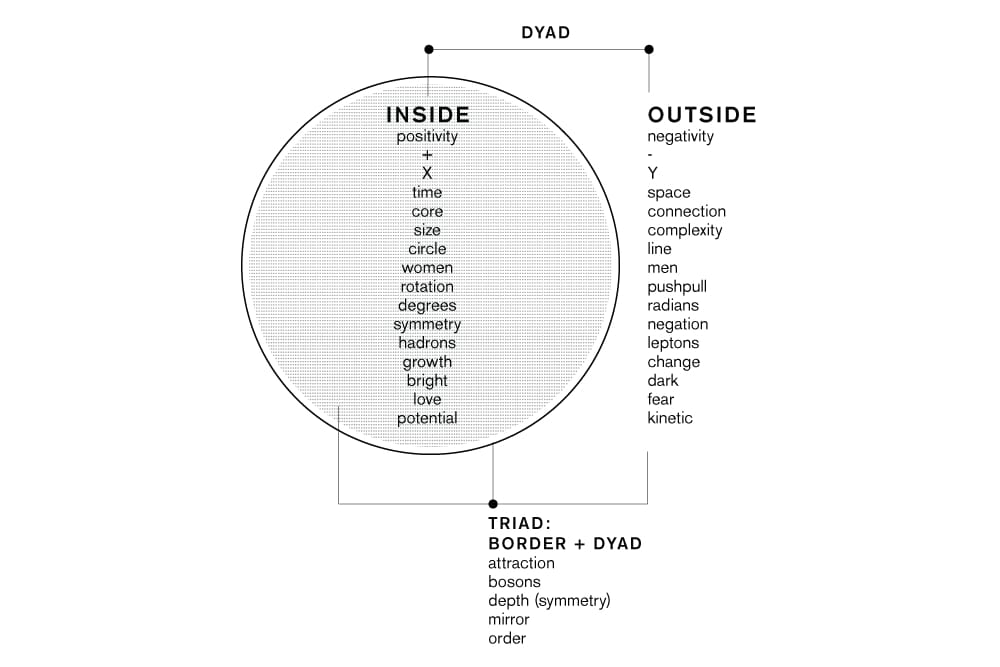# The Three Principles of Mindspace

In Thought Theory, The Three Principles are the foundational philosophies of Mindspace and the Universe. They represent the most basic Triad, that emerges from the most basic Dyad, that emerges from the most basic Monad (ie. the Circle).

These are the names of the principles:

• Symmetry
• Attraction
• Negation

In the hyper-dimensional structure they represent the three groups of dimensions:

• Physical dimensions
• Intelligence dimensions
• Wave dimensions

In the architecture of the circle they represent its three subsections:

• Inside
• Perimeter (Border)
• Outside

In physics they represent the three families of particles:

• Bosons
• Leptons

As functions of spacetime:

• Time
• 4 Forces of nature
• Space

They represent the perspectives of a circle:

• Circle [the top view]
• Rotation [force]
• Line [the side view]

In an economic model they represent its three phases:

• Growth
• Balance
• Change

They represent the most important integers and irrational constants:

• 1: Phi
• 3: Pi
• 2: e

As functions of the Attractive force they are:

• Size [a state]
• Order [a process]
• Complexity [a state]

They are found in the 3 Principles of HP’s Next-Generation Computer called ‘The Machine’:

• Ions store
• Photos communicate
• Electrons compute

They are also known as the 3 tools of Freemasonry:

• Compass
• Bible
• Square

Thought Theory uses an illustrative process called ‘conceptual layering’. It lets you re-describe seemingly different concepts as varying perspectives of the same base concept (the Circle).